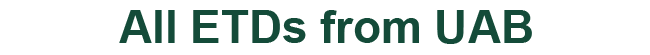### Analysis of Systems of First Order Linear Differential Equations with Non-Diagonalizable Matrices

#### Authors

Roman Shterenberg

Nikita Selinger

Nandor J Simanyi

Thesis

2022

#### Degree Name by School

Master of Science (MS) College of Arts and Sciences

#### Abstract

Finding solutions to differential equations is notoriously computationally intensive given that the number of operations required is heavily dependent on the algorithm being implemented. This is especially true for systems described by a matrix representation because as the eigenvalues of the system approach non-uniqueness, any approximations made by the program looking for the solution can lead to undesirable precision errors when calculating the final solution. In this document, two algorithms are compared which deal with this issue of stability which can greatly affect the decision to use one method over the other. In the method of solving the system by means of matrix diagonalization can be easily found given distinct eigenvalues; however, problems come up as the eigenvalues of the system converge as this leads to undefined entries in the inverse eigenvector matrix. The Putzer algorithm, however, sidesteps these singularities, resulting in a method that produces approximations independent of the angle between the eigenvectors of the system.

COinS